# Tổng hợp Đề thi Violympic Toán Tiếng Anh lớp 3 tất cả các vòng CÓ ĐÁP ÁN (3)

1 1.578

## Đề thi giải Toán Tiếng Anh trên mạng lớp 3 có đáp án

Tài liệu tham khảo Tổng hợp Đề thi Violympic Toán Tiếng Anh lớp 3 tất cả các vòng CÓ ĐÁP ÁN (3) sẽ giúp bạn thực hành giải các bài tập Tiếng Anh, phát triển kĩ năng giải bài trắc nghiệm, đồng thời ôn tập lại những kiến thức để chuẩn bị tốt cho kì thi. Chúc quý thầy cô và các em học sinh có được nguồn tư liệu hay mà mình đang tìm.

Đề thi Violympic Toán Tiếng Anh lớp 3 vòng 7 năm 2015 - 2016

Đề thi Violympic Toán Tiếng Anh lớp 3 vòng 8 năm 2015 - 2016 trực tuyến

Tổng hợp Đề thi Violympic Toán Tiếng Anh lớp 3 tất cả các vòng CÓ ĐÁP ÁN (1)

Tổng hợp Đề thi Violympic Toán Tiếng Anh lớp 3 tất cả các vòng CÓ ĐÁP ÁN (2)

Đề thi Violympic Toán Tiếng Anh lớp 3 vòng 10 năm 2015 - 2016

Exam number 1: Điền giá trị thích hợp vào chỗ chấm

Question 1: Fill in the blank with the suitable sign (>; =; <)

Compare: 4782 .............. 4827

Fill in the blank with the suitable number

Question 2: 9 hours 5 minutes = ............ minutes

Question 3: 5km 12m = .............. m

Question 4: Calculate: 2088 : 6 = ................

Question 5: Alan is five years old. His sister Bethany is seven years older than him. What is the sum of their ages?

Question 6: Anna is reading a book that has 192 pages. Mary is reading a book that has three times as many pages as Anna's book. How many pages does Mary's book have?

Question 7: There are 3 bags of beans. Each bag contains 78 beans. How many beans are there in all?

Question 8: If 63 breads are divided to 7 students, how many breads will each student have?

Question 9: I am an odd 2-digit number. The sum of my digits is 9. My greatest value is ................

Question 10: If you add 10000 to the largest 5- distinct digit number that can be formed by using the digits 5, 7, 6, 4, 2, then what is the resulting sum?

Exam number 2: Đi tìm kho báu

Question 1: Calculate: (3726 + 2954) x 0 = ...........

Question 2: Find m such that 9384 : m = 4.

Question 3: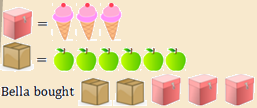She bought ...... less ice-creams than apples.

Question 4: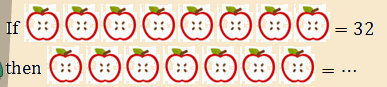Question 5: If the 2nd day of a month is Monday then the 27th day of the month is ........

a. Thursday

b. Friday

c. Wednesday

d. Tuesday

Exam number 3: Tìm cặp bằng nhau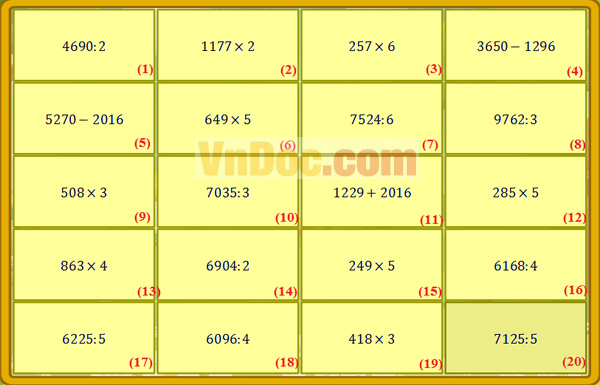Đáp án đề thi Violympic Toán Tiếng Anh lớp 3

Exam number 1: Điền giá trị thích hợp vào chỗ chấm

Question 1: <

Question 2: 545

Question 3: 5012

Question 4: 348

Question 5: 17

Question 6: 576

Question 7: 234

Question 8: 9

Question 9: 81

Question 10: 86542

Exam number 2: Đi tìm kho báu

Question 1: 0

Question 2: 2346

Question 3: 3

Question 4: 28

Question 5: b

Exam number 3: Tìm cặp bằng nhau

(1) = (10); (2) = (4); (3) = (16); (5) = (8); (6) = (11); (7) = (19); (9) = (18); (12) = (20); (13) = (14); (15) = (17)

Đề thi Violympic Toán Tiếng Anh lớp 3 vòng 9 năm 2015 - 2016

Exam number 1: Đỉnh núi trí tuệ

Question 1: Find x such that x : 6 = 42

a. 36            b. 7                 c. 48          d. 252

Question 2: Fill in the blank with a suitable operator 18 ........ 9 = 2

a. +             b. x                  c. :            d. -

Question 3: How many days are there in January?

a. 28            b. 29               c. 30           d. 31

Question 4: How long is segment AB in cm?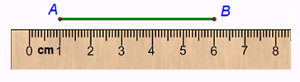a. 50              b. 60                c. 5                d. 6

Question 5: Find the value of x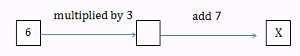a. 11               b. 9                 c. 126           d. 25

Question 6: Multiply 12 by 6 then subtract 25 from the product. The result is ............

a. 47                b. 27                c. 74             d. 97

Question 7: Divide 56 by 7 then subtract 5 from the quotient. The result is .............

a. 13                 b. 58              c. 3               d. 397

Question 8: By how many times is 15 greater than 5?

a. 10                 b. 20              c. 3                  d. 75

Question 9: Look at this table. Among these 4 people, who is the tallest?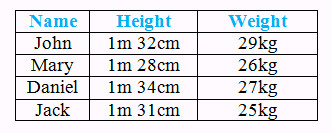a. Jack               b. John             c. Daniel           d. Mary

Question 10: Find the value of X: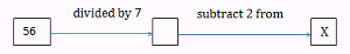a. 47                b. 10                 c. 6                 d. 65

Exam number 2: Cóc vàng tài ba

Question 1: There are 165 students in a grade 3, including 84 girls. How many boys are there in the grade 3?

a. 13860               b. 100                    c. 249            d. 81

Question 2: How do you write this number using digits? Seven hundred three.

a. 137                 b. 730                     c. 703            d. 173

Question 3: How many triangles are there?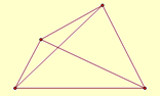a. 4                    b. 6                      c. 2             d. 8

Question 4: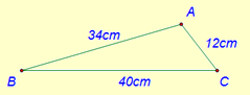The longest side of triangle ABC is ............ cm.

a. 40               b. 34                     c. 86           d. 12

Question 5: Danny is 31. Garrett is 4 years older than Bryce and 2 years older than Danny. How old is Bryce?

a. 33 years old                        b. 37 years old

c. 27 years old                        d. 29 years old

Question 6: Clayton is 5 years younger than Ruth. Jane is 36. Kimberly is 1 year younger than Jane and 5 years younger than Ruth. How old is Ruth?

a. 35 years old                         b. 37 years old

c. 41 years old                        d. 40 years old

Question 7: I am an even number. I am greater than 2 but less than 6. What number am I

a. 2                   b. 8                    c. 6              d. 4

Question 8: In a class, there are 40 students and the number of girls is 4 times the number of boys. How many boys are there?

a. 32                  b. 44                     c. 8               d. 10

Question 9: How many bricks like this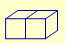are needed to make a wall like this?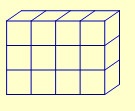a. 6                  b. 12                    c. 4                d. 3

Question 10: In my class, only 6 of 10 students were at school. How many were absent?

a. 7                  b. 10                     c. 6               d. 4

Exam number 3: Vượt chướng ngại vật

Question 1: How many green squares are there in the figure?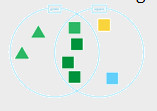Question 2: Coffee costs \$5 per pound. How much does 5 pounds of coffee cost?

Question 3: How many circles are there?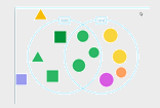Question 4: Look at the four angles marked on the picture of a house. Which angle is a right angle?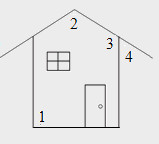Question 5: On Friday, 1250 people visited the zoo. The number of people visited the zoo on Saturday is three times as many as on Friday. How many people visited the zoo on Saturday?

Đáp án đề thi Violympic Toán Tiếng Anh lớp 3 vòng 9

Exam number 1: Đỉnh núi trí tuệ

Question 1: d

Question 2: c

Question 3: d

Question 4: c

Question 5: d

Question 6: a

Question 7: c

Question 8: c

Question 9: c

Question 10: c

Exam number 2: Cóc vàng tài ba

Question 1: d

Question 2: c

Question 3: d

Question 4: a

Question 5: d

Question 6: d

Question 7: d

Question 8: c

Question 9: a

Question 10: d

Exam number 3: Vượt chướng ngại vật

Question 1: 4

Question 2: 25

Question 3: 7

Question 4: 1

Question 5: 3750

Đánh giá bài viết
1 1.578